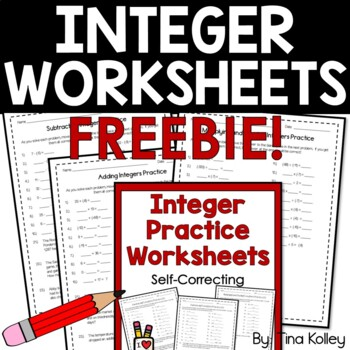1,043 Downloads

# Integers | Adding and Subtracting | Multiplying and Dividing | FREEGrade Levels
5th - 7th, Homeschool
Subjects
Standards
Resource Type
Formats Included
• PDF
Pages
9 pages
Share this resource

### Description

These no-prep, self-correcting Integer Practice Worksheets will have your students practicing adding, subtracting, multiplying, and dividing integers in no time. Your students will love the challenge of the puzzle-like questions, you will love the self-correcting aspect! Just download, print, and go! Perfect for homework, classwork, skill review, and collaborative work.

Total Pages
9 pages
Answer Key
Included
Teaching Duration
N/A
Report this Resource to TpT
Reported resources will be reviewed by our team. Report this resource to let us know if this resource violates TpT’s content guidelines.

### Standards

to see state-specific standards (only available in the US).
Understand that integers can be divided, provided that the divisor is not zero, and every quotient of integers (with non-zero divisor) is a rational number. If 𝘱 and 𝘲 are integers, then –(𝘱/𝘲) = (–𝘱)/𝘲 = 𝘱/(–𝘲). Interpret quotients of rational numbers by describing real-world contexts.
Understand that multiplication is extended from fractions to rational numbers by requiring that operations continue to satisfy the properties of operations, particularly the distributive property, leading to products such as (–1)(–1) = 1 and the rules for multiplying signed numbers. Interpret products of rational numbers by describing real-world contexts.
Apply and extend previous understandings of multiplication and division and of fractions to multiply and divide rational numbers.
Apply properties of operations as strategies to add and subtract rational numbers.
Understand subtraction of rational numbers as adding the additive inverse, 𝘱 – 𝘲 = 𝘱 + (–𝘲). Show that the distance between two rational numbers on the number line is the absolute value of their difference, and apply this principle in real-world contexts.

### Questions & Answers

Teachers Pay Teachers is an online marketplace where teachers buy and sell original educational materials.

More About Us